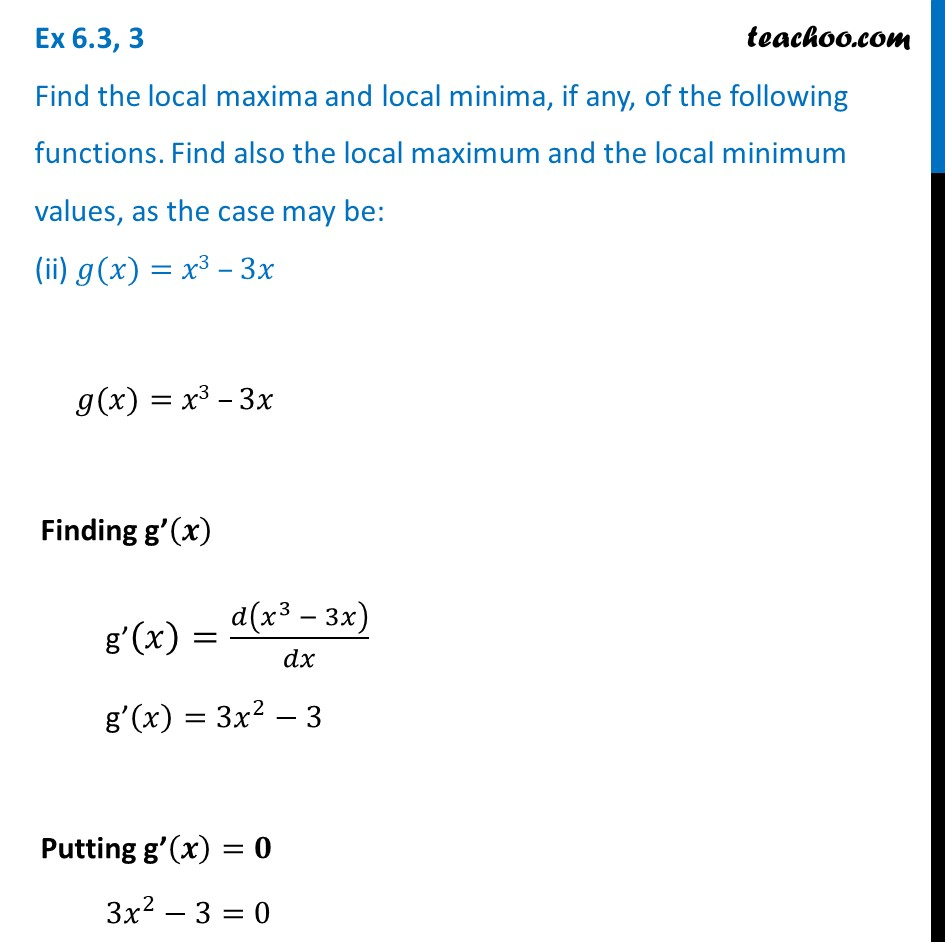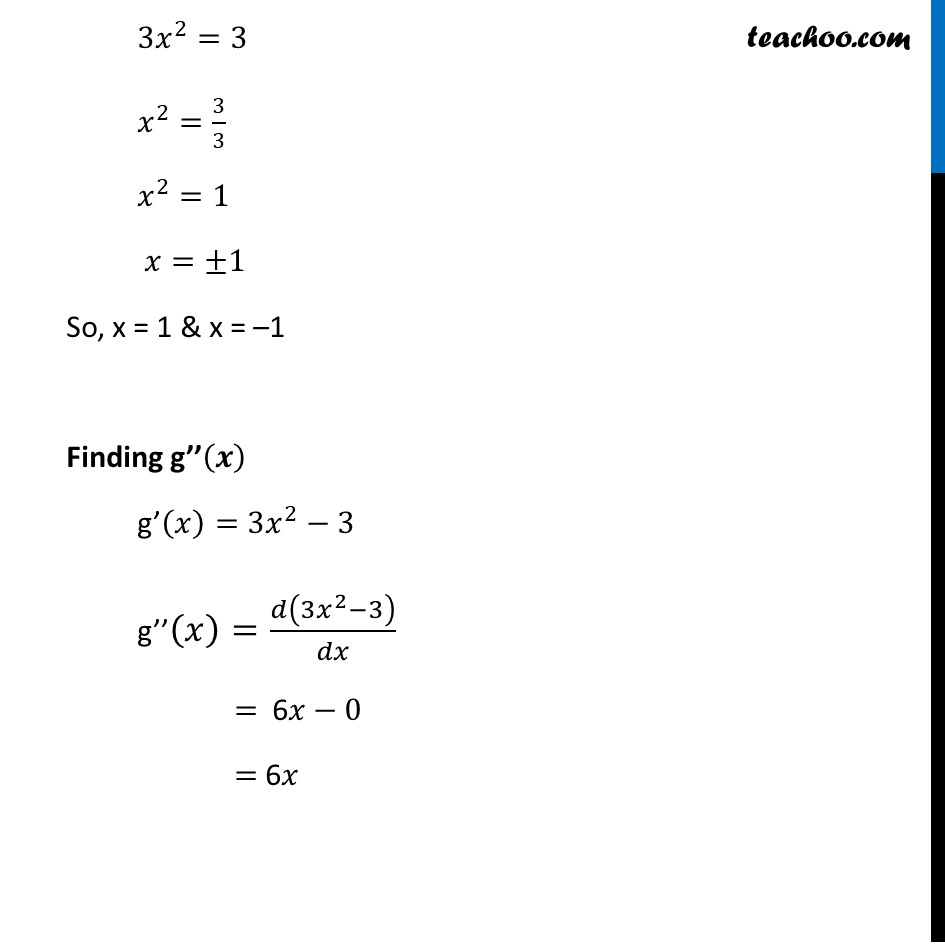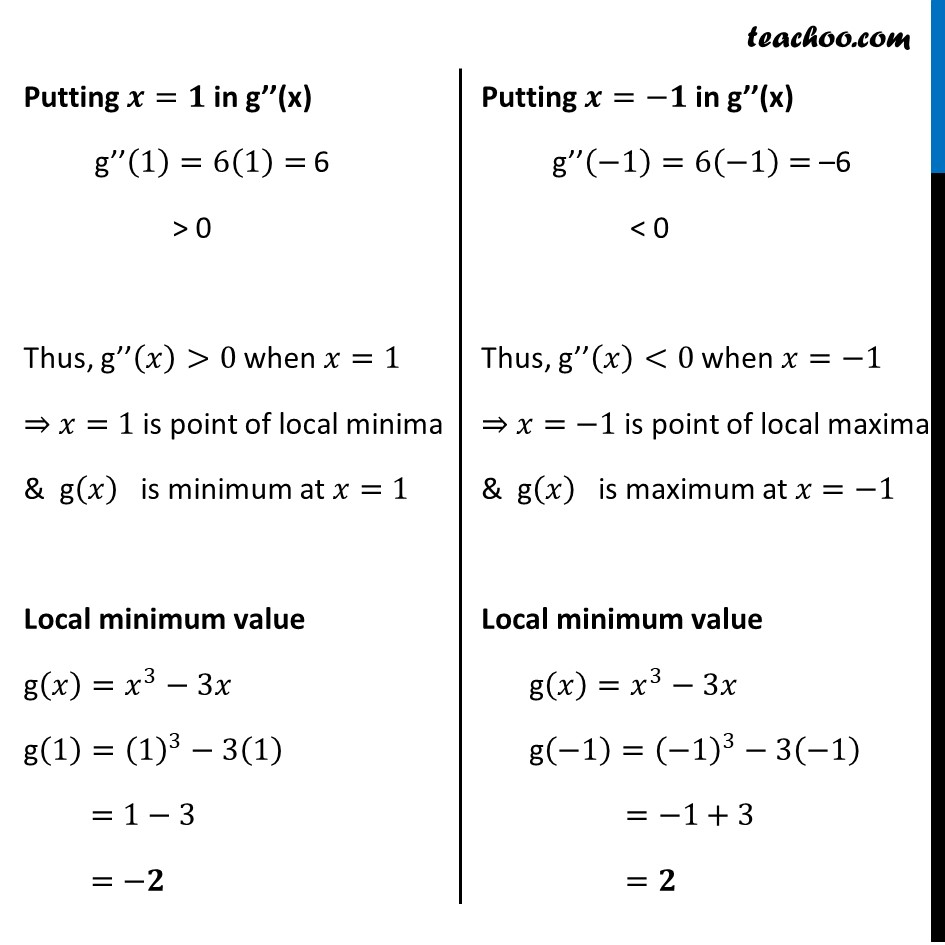Ex 6.3

Chapter 6 Class 12 Application of Derivatives
Serial order wiseLearn in your speed, with individual attention - Teachoo Maths 1-on-1 Class

### Transcript

Ex 6.3, 3 Find the local maxima and local minima, if any, of the following functions. Find also the local maximum and the local minimum values, as the case may be: (ii) 𝑔(𝑥)=𝑥3 –3𝑥𝑔(𝑥)=𝑥3 –3𝑥 Finding g’(𝒙) g’(𝑥)=𝑑(𝑥^3 − 3𝑥)/𝑑𝑥 g’(𝑥)=3𝑥^2−3 Putting g’(𝒙)=𝟎 3𝑥^2−3=0 3𝑥^2=3 𝑥^2=3/3 𝑥^2=1 𝑥=±1 So, x = 1 & x = –1 Finding g’’(𝒙) g’(𝑥)=3𝑥^2−3 g’’(𝑥)=𝑑(3𝑥^2−3)/𝑑𝑥 = 6𝑥−0 = 6𝑥 Putting 𝒙=𝟏 in g’’(x) g’’(1)=6(1)= 6 > 0 Thus, g’’(𝑥)>0 when 𝑥=1 ⇒ 𝑥=1 is point of local minima & g(𝑥) is minimum at 𝑥=1 Local minimum value g(𝑥)=𝑥^3−3𝑥 g(1)=(1)^3−3(1) =1−3 =−𝟐 Putting 𝒙=−𝟏 in g’’(x) g’’(−1)=6(−1)= –6 < 0 Thus, g’’(𝑥)<0 when 𝑥=−1 ⇒ 𝑥=−1 is point of local maxima & g(𝑥) is maximum at 𝑥=−1 Local minimum value g(𝑥)=𝑥^3−3𝑥 g(−1)=(−1)^3−3(−1) =−1+3 =𝟐